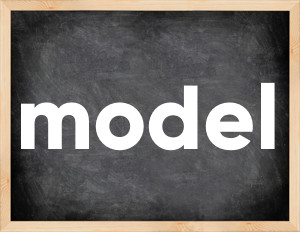# Model past tenseThe English verb 'model' is pronounced as [ˈmɑːdl].
Related to: regular verbs.
3 forms of verb model: Infinitive (model), Past Simple - (modeled), Past Participle - (modeled).

## Here are the past tense forms of the verb model

👉 Forms of verb model in future and past simple and past participle.
❓ What is the past tense of model.

## Model: Past, Present, and Participle Forms

Base Form Past Simple Past Participle
model [ˈmɑːdl]

modeled [ˈmɑːdəld]

modeled [ˈmɑːdəld]

## What are the 2nd and 3rd forms of the verb model?

🎓 What are the past simple, future simple, present perfect, past perfect, and future perfect forms of the base form (infinitive) 'model'?

### Learn the three forms of the English verb 'model'

• the first form (V1) is 'model' used in present simple and future simple tenses.
• the second form (V2) is 'modeled' used in past simple tense.
• the third form (V3) is 'modeled' used in present perfect and past perfect tenses.

## What are the past tense and past participle of model?

The past tense and past participle of model are: model in past simple is modeled, and past participle is modeled.

### What is the past tense of model?

The past tense of the verb "model" is "modeled", and the past participle is "modeled".

### Verb Tenses

Past simple — model in past simple modeled (V2).
Future simple — model in future simple is model (will + V1).
Present Perfect — model in present perfect tense is modeled (have/has + V3).
Past Perfect — model in past perfect tense is modeled (had + V3).

### model regular or irregular verb?

👉 Is 'model' a regular or irregular verb? The verb 'model' is regular verb.

## Examples of Verb model in Sentences

•   You have to be careful to model bad behavior for the children (Present Simple)
•   The climatology was modelled in adequate way (Past Simple)
•   We want to continue to model this process (Present Simple)
•   He wants me to model (Present Simple)
•   She modeled for him in a few photoshoots (Past Simple)
•   She models for a living (Present Simple)
•   We can model this by using linear regression (Present Simple)
•   He models himself after Hollywood actors (Present Simple)
•   Our small firm earns money by modeling ships (Present Simple)
•   Before we embark on a journey, we have to carefully model the process (Present Simple)

Along with model, words are popular page and feel up to.

Verbs by letter: , , , , , , , , , , , , , , , , , , , , , , , , .